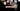# Leetcode | Solution of Create Target Array in the Given Order in JavaScript

May 14th, 2020
|

In this post, we will solve Create Target Array in the Given Order from leetcode and compute it's time and space complexities. Let's begin.

# Problem Statement

The question can be found at leetcode Create Target Array in the Given Order problem.

The problem states that we are given two arrays, `nums`, and `index`. For each position `i` in `nums` and `index`, we need to create an array whose value at position `index[i]` is `nums[i]`.

``  Something like target[index[i]] = nums[i]``

# Solution

There are multiple ways to solve this. You can iterate over the index array and for every value in the index array, you can push values to target array at position `index[i]` i.e. `nums[i]`. It requires a lot of shifting of elements in the array where you can use the `slice` method to do it in one line.

For our solution, we will use reduce method and the spread operator but the logic is still same. It just keeps the program simple to read.

``````var createTargetArray = function(nums, index) {

return index.reduce((acc,el,idx) => {
return [...acc.slice(0,el), nums[idx], ...acc.slice(el)]
},[])
};``````

The code looks a bit fancy, but it is just looping over the index array. For every index, we break the array into two parts. The one before the index, and after the index. In the middle of both, we insert our value and return the whole thing

Here are the stats on submission

``````Status: Accepted
Runtime: 56ms
Memory: 37.1MB``````

## Time and space complexity

### Time complexity

We are looping over the index array once, so time complexity would be O(n).

### Space complexity

We are not using any extra space. So space complexity is constant, O(1).

# Summary

So, we solved the Create Target Array in the Given Order problem by using reduce and spread operator and calculated the time and space complexities.

I hope you enjoyed solving this question. This is it for this one, complete source code for this post can be found on my Github Repo. Will see you in the next one.